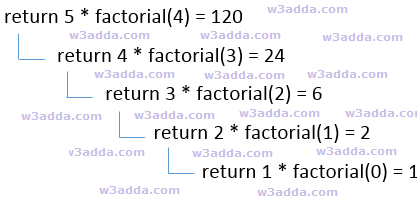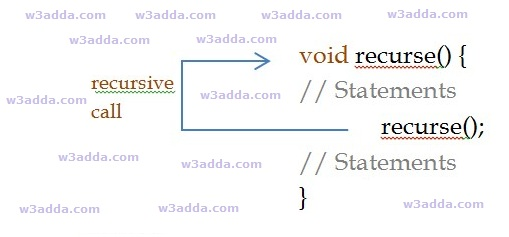# C++ Recursion

In this tutorial you will learn about the C++ Recursion and its application with practical example.

## What Is Recursion?

Recursion is the process where a function calls itself as its subroutine in order to solve a complex iterative task by dividing it into sub tasks. Any function which calls itself recursively is called recursive function, and the process of calling a function by itself is called recursion. Recursion leads to several number of iterative calls to the same function, however, it is important to have a base case to terminate the recursion.

Recursion is an efficient approach to solve a complex mathematical computation task by dividing it into sub tasks. This approach of solving a problem is called as Divide and Conquer. In programming, it allows programmers to divide a complex problem into sub tasks and to solve them individually to reach final solution.

Any problem that can be solved recursively, can also be solved iteratively but recursion is considered as more efficient method of programming as it requires the least amount of code to perform same complex task. Although recursion is not recommended for all problems, but it is best suited for some problems like sorting, searching, Inorder/Preorder/Postorder Tree Traversals, DFS of Graph algorithms. However, recursion must be implemented carefully, otherwise it may lead to an infinite loop if no base condition is met that will terminate the function.

Note :- Recursive function must have a valid terminating condition or base case, otherwise it will leads to an infinite loop.

## How recursion works?

To understand how recursion works lets have one of the popular example of recursion. In this example we will calculate the factorial of n numbers. The factorial of n numbers is expressed as a series of repetitive multiplication as shown below:

Example :-

dsfsdf## Recursive Function

A recursive function is not much different from any other function, basically a function calling itself directly or indirectly is known as recursive function. A recursive function repeats itself several times, in order to compute or return final output. Recursive functions are quite common in computer programing as they allow programmers to write efficient programs with minimal code.

## Characteristics of a recursive function

• A recursive function is a function which calls itself.
• The speed of a recursive program is slower because of stack overheads.
• A recursive function must have terminating conditions or base case, and recursive expressions.

Below is general syntax of a recursive function –

Syntax:-

Example:-## Types of Recursion

Recursive function can be of following two types based on the way it call –

Direct Recursion :- When a function calls itself directly is called as direct recursive function and this type of recursion is said to be direct recursion.

Indirect Recursion :- When a function calls itself indirectly from other function then this function is called as indirect recursive and this type of recursion is said to be indirect recursion.

## Memory Allocation In Recursion

Memory allocation for recursive function is no different from any other function. When a recursive function is called, it is allocated with a single memory block in a stack. This memory block holds up the required memory space for successful execution of the function and to hold all of the local, automatic and temporary variables. For each of the recursive call it pushes a separate stack frame in same manner. If a recursive function gets called 5 times, then there will 5 stack frames corresponding to each of the recursive call. When the recursive call ends, the new stack frame is discarded and function starts to return its value to the function in previous stack frame and then stack gets popped out in same order it was pushed and memory is de-allocated, this process continues till it reach to the final call. At the end recursion, it returns the final result value and finally the stack gets destroyed and memory is freed up. If the recursion fails to reach a base case, then the stack will rapidly be exhausted leading to Stack Overflow crash.

• It requires few variables which make program clean.
• It shorten the complex and nested code.

• It is hard to debug recursive function.
• It is tough to understand the logic of a recursive function.
• It can cause infinite loop or unexpected results if not written correctly.
• Recursive program has greater memory space requirements than iterative program.
• Recursive programs are slower than non recursive programs.

Example:-

## Factorial of a number using Recursion

Output:-Suppose value of n=5, since n is greater than 1, the statement:

n* fact(n-1);

will be executed with n=5 i.e.

5* fact(5-1);

will be evaluated. As you can see this statement again calls factorial function with value n-1 which will return value:

4*fact(4-1);

This recursive calling process continues until value of n is equal to 1 and when n is equal to 1 it returns 1 and execution of this function stops. We can review the series of recursive call as follow:

f = 5* fact(5-1);

f = 5*4* fact(4-1);

f = 5*4*3* fact(3-1);

f = 5*4*3*2* fact(2-1);

f = 5*4*3*2*1;

f = 120;

## What is tail recursion?

When a recursive function has its recursive call as last statement to be executed then this form of recursion is called as tail recursion and function is tail recursive. Tail recursive functions are considered better than non tail recursive functions as it can be optimized by compiler, since recursive call is the last statement, hence there is no need to keep track of function’s current state in stack frame.

Example:-

In this tutorial we have learn about the C++ Recursion and its application with practical example. I hope you will like this tutorial.